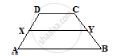# In the Below Fig. Abcd is a Trapezium in Which Ab || Dc and Dc = 40 Cm and Ab = 60 Cm. If X and Y Are Respectively, the Mid-points of Ad and Bc, Prove That: (I) Xy = 50 Cm(Ii) Dcyx is a Trapezium - Mathematics

In the below fig. ABCD is a trapezium in which AB || DC and DC = 40 cm and AB = 60
cm. If X and Y are respectively, the mid-points of AD and BC, prove that:
(i) XY = 50 cm
(ii) DCYX is a trapezium
(iii) ar (trap. DCYX) =9/11ar (trap. (XYBA))

#### Solution(1) Join DY and produce it to meet AB produced at P
In Δ' s BYP  and CYDwe have

∠BYP =(∠CYD)           [Vertical opposite angles]

∠DCY ∠ (∠CYD)           [∴ DC || AP ]

And By = CY

So, by ASA congruence criterion, we have

Δ BYP ≅ CYD

⇒ DY  =YP and DC = BP

⇒ y is the midpoint of DP
Also, x is the midpoint of AD

∴ XY || AP and XY = 1/2AD

⇒ xy = 1/2 ( AB + BD)

⇒ xy = 1/2 (BA + DC )⇒ xy  = 1/2 ( 60 + 40 )

(2) We have

XY  || AP

⇒ XY || AB and AB  || DC         [As proved above]

⇒  XY ||  DC

⇒ DCY is a trapezium

(3)   Since x and y are the midpoint of DA and CB respectively
∴Trapezium DCXY and ABYX are of the same height say hm

Now

ar (Trap DCXY) =1/2(DC + XY) × h

= 1/2   (50 + 40) hcm^2  = 45  hcm^2

⇒ ar (trap  ABXY) = 1/2 (AB + XY) xx h = 1/2 (60 + 50) hm^3

⇒ar (trap  ABXY) = 1/2 (AB + XY) xx h = 1/2 (60 + 50) hm^2

= 55 cm2

 (ar trap (YX))/(ar trap (ABYX)) = (45h )/ (55h)= 9 /11

⇒ ar (trap DCYX) =9/11 ar (trap ABXY)

Concept: Corollary: A rectangle and a parallelogram on the same base and between the same parallels are equal in area.
Is there an error in this question or solution?

#### APPEARS IN

RD Sharma Mathematics for Class 9
Chapter 14 Areas of Parallelograms and Triangles
Exercise 14.3 | Q 23 | Page 47

Share